# SSAT Upper Level Math : How to find the nth term of an arithmetic sequence

## Example Questions

2 Next →

### Example Question #41 : Sequences And Series

The lengths of the sides of ten squares form an arithmetic sequence. One side of the smallest square measures eight inches; one side of the second-smallest square measures one foot.

Give the area of the largest square.

784 square inches

484 square inches

1,936 square inches

2,304 square inches

576 square inches

1,936 square inches

Explanation:

Let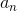be the lengths of the sides of the squares in inches.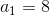and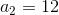, so their common difference is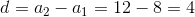The arithmetic sequence formula is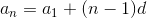The length of a side of the largest square - square 10 - can be found by substituting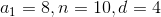: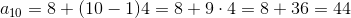The largest square has sides of length 44 inches, so its area is the square of this, or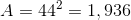square inches.

### Example Question #42 : Sequences And Series

An arithmetic sequence begins as follows: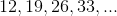Give the thirty-second term of this sequence.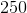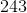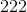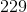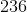Explanation:

Theth term of an arithmetic sequence with initial term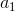and common differenceis defined by the equationThe initial term in the given sequence is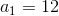;

the common difference is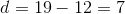;

We are seeking term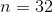.

This term is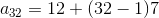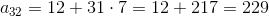### Example Question #43 : Sequences And Series

An arithmetic sequence begins as follows: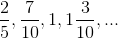Give the thirty-third term of this sequence.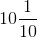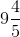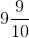The correct answer is not given among the other four responses.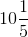The correct answer is not given among the other four responses.

Explanation:

Theth term of an arithmetic sequence with initial termand common differenceis defined by the equation.

The initial term in the given sequence is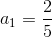;

the common difference is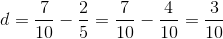.

We are seeking term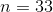.

Therefore,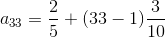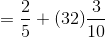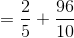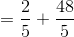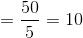,

which is not among the choices.

2 Next →

### All SSAT Upper Level Math Resources